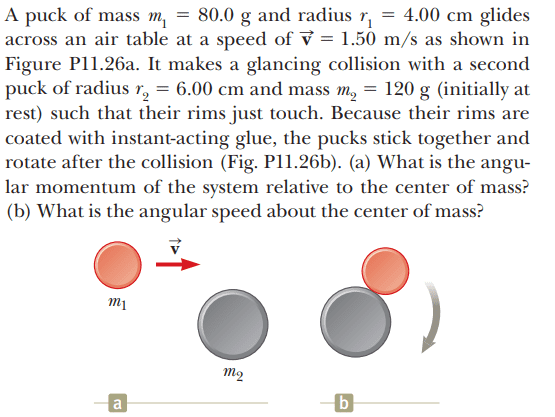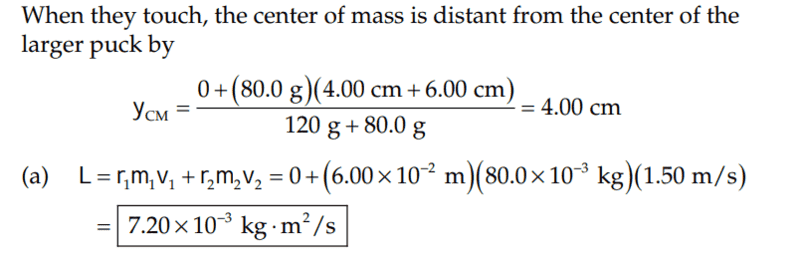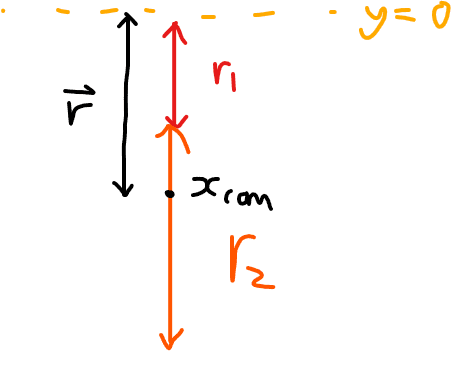# Air table puck collision

Callumnc1
Homework Statement:
Relevant Equations:
For part(a),The solution is,However, I made a mistake somewhere in my working below and I'm not sure what it is. Does anybody please know? Thank you!

Here is a not too scale diagram at the moment of the collision,## \vec L = \vec r \times \vec p ##
## \vec L = -y_{com}\hat j \times m_1v\hat i ##
## \vec L = y_{com}m_1v\hat k ##
## \vec L = \frac {m_2m_1v(r_1 +r_2)}{m_1 + m_2}\hat k ##

#### Attachments

Last edited:

Homework Helper
Gold Member
For part (a) please show what the distance ##y_1## from the CoM to the center of puck 1 is and how you got it in symbolic form.

•Callumnc1
Callumnc1
For part (a) please show what the distance ##y_1## from the CoM to the center of puck 1 is and how you got it in symbolic form.

I assume that the COM of each puck is at the geometric center.

Choosing the center of ##m_1## as the origin where ##y = 0## and let ##r_3## be the vertical distance from ## y = 0## to the COM of ##m_2##.

## y_1 = y_{com} = \frac {m_1(0) + m_2r_3} {m_1 + m_2} ##

## y_1 = \frac {m_2(r_1 + r_2)} {m_1 + m_2} ##

Then substituting in values,

## y_1 = \frac {0.12(0.1)} {0.2} ##
## y_1 = 0.06 m ##

Also please see post #1, I missed some of the notations so I have edited it.

Many thanks!

Callumnc1
Thank you for your help @kuruman! I see now how they got their answer. I think I got confused because the solutions calculated the ##y_{com}## from a different point. Good idea to use ##y_1## notation for calculations of CoM with respect to different origins!

Many thanks!